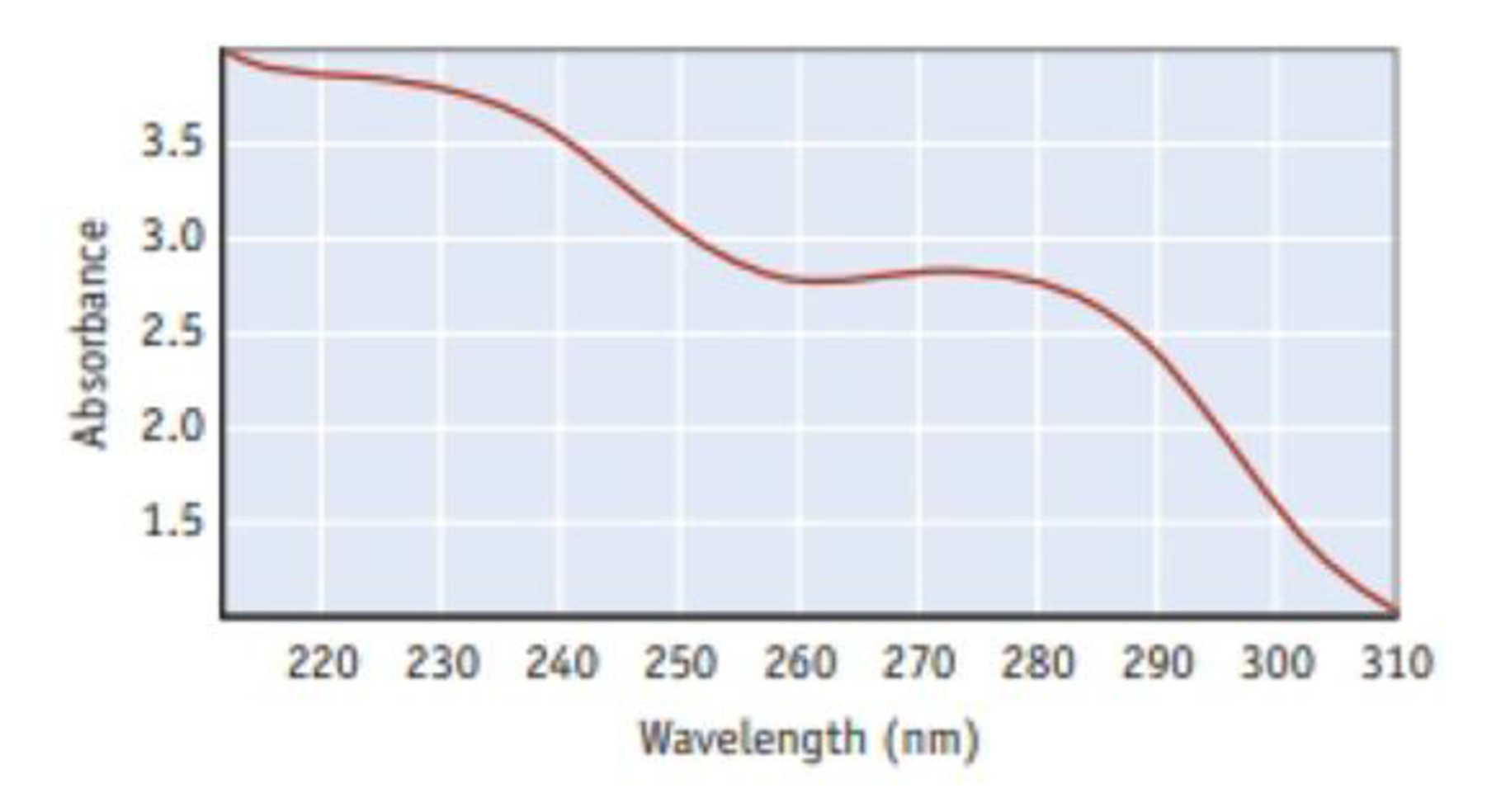# The spectrum shown here is for aspirin. The vertical axis is the amount of light absorbed, and the horizontal axis is the wavelength of incident light (in nm). (For more on spectrophotometry, see Section 4.9) What is the frequency of light with a wavelength of 278 nm? What is the energy of one mole of photons with ʎ = 278 nm? What region of the electromagnetic spectrum is covered by the spectrum above? Knowing that aspirin only absorbs light in the region depicted by this spectrum. What is the color of aspirin?### Chemistry & Chemical Reactivity

9th Edition
John C. Kotz + 3 others
Publisher: Cengage Learning
ISBN: 9781133949640

#### Solutions

Chapter
Section### Chemistry & Chemical Reactivity

9th Edition
John C. Kotz + 3 others
Publisher: Cengage Learning
ISBN: 9781133949640
Chapter 6, Problem 72IL
Textbook Problem
135 views

## The spectrum shown here is for aspirin. The vertical axis is the amount of light absorbed, and the horizontal axis is the wavelength of incident light (in nm). (For more on spectrophotometry, see Section 4.9)What is the frequency of light with a wavelength of 278 nm? What is the energy of one mole of photons with ʎ = 278 nm? What region of the electromagnetic spectrum is covered by the spectrum above? Knowing that aspirin only absorbs light in the region depicted by this spectrum. What is the color of aspirin?

Interpretation Introduction

Interpretation: The frequency and energy per mole of photons has to be calculated. The region of absorption spectrum and colour of aspirin has to be identified.

Concept introduction:

• Electromagnetic radiations are a type of energy surrounding us. They are of different types like radio waves, IR, UV, X-ray etc.
• Visible light lies in the wavelength of 400nm to 700nm• Planck’s equation,

E=where, E=energyh=Planck'sconstantν=frequency

The energy increases as the wavelength of the light decrease. Also the energy increases as the frequency of the light increases.

• The frequency of the light is inversely proportional to its wavelength.

ν=cλwhere, c=speedoflightν=frequencyλ=wavelength

• Electromagnetic radiations are a type of energy surrounding us. They are of different types like radio waves, IR, UV, X-ray etc.
• Ultraviolet radiation lies between 200nm to 400nm wavelength region.

### Explanation of Solution

Given,

The wavelength of light is 278 nm=2.78 ×107m.

• The frequency per mole of photons is calculated,

ν=cλ

Substituting the values

ν=2.998×108m/s2.78 ×107m=1.078×1015s1

The frequency per mole of photons is 1.078×1015s1

• The energy per mole of photons is calculated.

Energyperphoton==6

### Still sussing out bartleby?

Check out a sample textbook solution.

See a sample solution

#### The Solution to Your Study Problems

Bartleby provides explanations to thousands of textbook problems written by our experts, many with advanced degrees!

Get Started

Find more solutions based on key concepts
Which weighs more, 0.001 g of water or 1 mg of water?

Introductory Chemistry: A Foundation

What behaviors would be most helpful in preventing osteoporosis?

Understanding Nutrition (MindTap Course List)

For managing type 2 diabetes, regular physical activity can help by redistributing the body's fluids. T F

Nutrition: Concepts and Controversies - Standalone book (MindTap Course List)

How do a solute, a solvent, and a solution differ?

Biology: The Dynamic Science (MindTap Course List)

What is the difference between a hypothesis , theory, and a law?

Horizons: Exploring the Universe (MindTap Course List)

What parts of the hydrologic cycle take millions of years to complete?

Oceanography: An Invitation To Marine Science, Loose-leaf Versin

Why is the following situation impossible? An ideal gas undergoes a process with the following parameters: Q = ...

Physics for Scientists and Engineers, Technology Update (No access codes included)